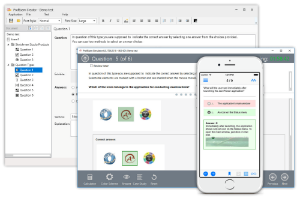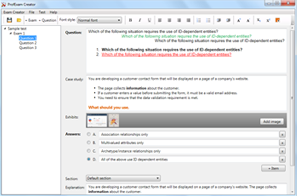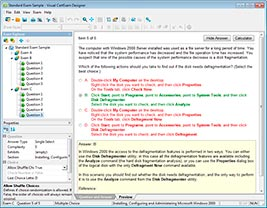## File Info

 Exam Java SE 8 Programmer II Number 1z0-809 File Name Java SE 8 Programmer II.passcertification.1z0-809.2020-01-30.1e.117q.vcex Size 4.2 Mb Posted January 30, 2020 Downloads 82

### How to open VCEX & EXAM Files?

Files with VCEX & EXAM extensions can be opened by ProfExam Simulator.

Coupon: EXAMFILESCOM
With discount: 20%

## Demo Questions

Question 1
Given the definition of the Vehicle class:
Class Vehicle {
int distance;
Vehicle (int x) {
this distance = x;
}
public void increSpeed(int time)   {
int timeTravel = time;                 //line n1
//line n3
class Car {
int value = 0;
public void speed () {
value = distance /timeTravel;    //line n2
System.out.println (“Velocity with new speed”+value+”kmph”);
}
}
speed();                        //line n3
}

and this code fragment:
Vehicle v = new Vehicle (100);
v.increSpeed(60);
What is the result?

• A: Velocity with new speed 1 kmph
• B: A compilation error occurs at line n1.
• C: A compilation error occurs at line n2.
• D: A compilation error occurs at line n3.

Question 2
Given the code fragment:
List<Integer> values = Arrays.asList (1, 2, 3);
values.stream ()
.map(n -> n*2)        //line n1
.peek(System.out::print)    //line n2
.count();
What is the result?

• A: 246
• B: The code produces no output.
• C: A compilation error occurs at line n1.
• D: A compilation error occurs at line n2.

Question 3
Given:
class Sum extends RecursiveAction   {                 //line n1
static final int THRESHOLD_SIZE  = 3;
int stIndex, lstIndex;
int [ ] data;
public Sum (int [ ]data, int start, int end)   {
this.data = data;
this stIndex = start;
this. lstIndex = end;
}
protected void compute ( )    {
int sum = 0;
if (lstIndex – stIndex <= THRESHOLD_SIZE)  {
for (int i = stIndex; i < lstIndex; i++)   {
sum += data [i];
}
System.out.println(sum);
} else {
new Sum (data, stIndex + THRESHOLD_SIZE, lstIndex).fork( );
new Sum (data, stIndex,
Math.min  (lstIndex, stIndex + THRESHOLD_SIZE)
).compute ();
}
}

and the code fragment:
ForkJoinPool fjPool = new ForkJoinPool ( );
int data [ ] = {1, 2, 3, 4, 5, 6, 7, 8, 9, 10}
fjPool.invoke (new Sum (data, 0, data.length));
and given that the sum of all integers from 1 to 10 is 55.
Which statement is true?

• A: The program prints several values that total 55.
• B: The program prints 55.
• C: A compilation error occurs at line n1.
• D: The program prints several values whose sum exceeds 55.

Question 4
Given the content of Operator.java, EngineOperator.java, and Engine.java files:and the code fragment:What is the result?

• A: The Engine.java file fails to compile.
• B: The EngineOperator.java file fails to compile.
• C: The Operator.java file fails to compile.
• D: ON OFF

Question 5
Given the code fragment:
Path file = Paths.get (“courses.txt”);
// line n1
Assume the courses.txt is accessible.
Which code fragment can be inserted at line n1 to enable the code to print the content of the courses.txt file?

• A: List<String> fc = Files.list(file);
fc.stream().forEach (s - > System.out.println(s));
• B: Stream<String> fc = Files.readAllLines (file);
fc.forEach (s - > System.out.println(s));
• C: List<String> fc = readAllLines(file);
fc.stream().forEach (s - > System.out.println(s));
• D: Stream<String> fc = Files.lines (file);
fc.forEach (s - > System.out.println(s));

Question 6
Given the code fragment:
public void recDelete (String dirName) throws IOException   {
File [ ] listOfFiles = new File (dirName) .listFiles();
if (listOfFiles ! = null && listOfFiles.length >0)   {
for (File aFile : listOfFiles)   {
if (aFile.isDirectory ())    {
recDelete (aFile.getAbsolutePath ());
}    else   {
if (aFile.getName ().endsWith (“.class”))
aFile.delete ();
}
}
}

Assume that Projects contains subdirectories that contain .class files and is passed as an argument to the recDelete () method when it is invoked.
What is the result?

• A: The method deletes all the .class files in the Projects directory and its subdirectories.
• B: The method deletes the .class files of the Projects directory only.
• C: The method executes and does not make any changes to the Projects directory.
• D: The method throws an IOException.

Question 7
Given the code fragments:
4. void doStuff() throws ArithmeticException, NumberFormatException, Exception   {
5.     if (Math.random() >-1 throw new Exception (“Try again”);
6. }
and
24. try {
25.     doStuff ( ):
26. } catch (ArithmeticException | NumberFormatException | Exception e)  {
27.     System.out.println (e.getMessage()); }
28. catch (Exception e)    {
29.     System.out.println (e.getMessage()); }
30. }
Which modification enables the code to print Try again?

• A: Comment the lines 28, 29 and 30.
• B: Replace line 26 with:
} catch (Exception | ArithmeticException | NumberFormatException e) {
• C: Replace line 26 with:
} catch (ArithmeticException | NumberFormatException e) {
• D: Replace line 27 with:
throw e;

Question 8
Given the definition of the Country class:
public class country {
public enum Continent {ASIA, EUROPE}
String name;
Continent region;
public Country (String na, Continent reg)   {
name = na, region = reg;
}
public String getName () {return name;}
public Continent getRegion () {return region;}

and the code fragment:
List<Country> couList = Arrays.asList (
new Country (“Japan”, Country.Continent.ASIA),
new Country (“Italy”, Country.Continent.EUROPE),
new Country (“Germany”, Country.Continent.EUROPE));
Map<Country.Continent, List<String>> regionNames = couList.stream ()
.collect(Collectors.groupingBy (Country ::getRegion,
Collectors.mapping(Country::getName, Collectors.toList()))));
System.out.println(regionNames);

• A: {EUROPE = [Italy, Germany], ASIA = [Japan]}
• B: {ASIA = [Japan], EUROPE = [Italy, Germany]}
• C: {EUROPE = [Germany, Italy], ASIA = [Japan]}
• D: {EUROPE = [Germany], EUROPE = [Italy], ASIA = [Japan]}

Question 9
Given:
class Book {
int id;
String name;
public Book (int id, String name)  {
this.id = id;
this.name = name;
}
public boolean equals (Object obj) {           //line n1
boolean output = false;
Book b = (Book) obj;
if (this.name.equals(b name))}
output = true;
}
return output;
}

and the code fragment:
Book b1 = new Book (101, “Java Programing”);
Book b2 = new Book (102, “Java Programing”);
System.out.println (b1.equals(b2));                 //line n2
Which statement is true?

• A: The program prints true.
• B: The program prints false.
• C: A compilation error occurs. To ensure successful compilation, replace line n1 with:
boolean equals (Book obj)  {
• D: A compilation error occurs. To ensure successful compilation, replace line n2 with:
System.out.println (b1.equals((Object) b2));

Question 10
Given the content of /resourses/Message.properties:
welcome1=”Good day!”
and given the code fragment:
Properties prop = new Properties ();
FileInputStream fis = new FileInputStream (“/resources/Message.properties”);
System.out.println(prop.getProperty(“welcome1”));
System.out.println(prop.getProperty(“welcome2”, “Test”));//line n1
System.out.println(prop.getProperty(“welcome3”));
What is the result?

• A: Good day!
Test
followed by an Exception stack trace
• B: Good day!
followed by an Exception stack trace
• C: Good day!
Test
null
• D: A compilation error occurs at line n1.

CONNECT US##### PROFEXAM WITH A 20% DISCOUNT

You can buy ProfExam with a 20% discount..

HOW TO OPEN VCEX AND EXAM FILES

Use ProfExam Simulator to open VCEX and EXAM filesHOW TO OPEN VCE FILES

Use VCE Exam Simulator to open VCE files